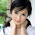## Monday, September 2, 2013

### Differential Equations #9: Solving x'(t) = f(y(t)), y'(t) = g(x(t))

Solving
x'(t) = f(y(t))
y'(t) = g(x(t))

Sometimes using algebra can be more efficient than using Laplace transforms in solving systems of differential equations.

Procedure - two paths we can take:

1. Take the derivative of x', x" = f'(y)
2. Solve for y' and equate it to g(x).
3. Solve for x(t).
4. Solve for y(t).

OR

1. Take the derivative of y', y" = g'(x)
2. Solve for x' and equate it to f(y).
3. Solve for y(t).
4. Solve for x(t).

Let's take some examples and see how this works. In all examples presented, x and y are functions of t.

1.
x'= 2y
y'= -2x

x" = 2y'

Solving for y':
y' = x"/2

Equate to g(x):
x"/2 = -2x
0 = x"/2 - 2x
0 = x" - 4x

We have a homogenous second order differential equation.

Characteristic Equation: λ^2 + 4 = 0
Roots: λ = ± 2i

Hence
x = C1 cos(2t) + C2 sin(2t)

Note x' = 2y.
y = x'/2
y = [d/dt C1 cos(2t) + C2 sin(2t) ] /2
y = -C1 sin(2t) + C2 cos(2t)

Solution:
x = C1 cos(2t) + C2 sin(2t)
y = -C1 sin(2t) + C2 cos(2t)

The practical approach to this type of problem is to end up working with a homogenous second order differential equation, if possible.

2.
x' = y + 1
y' = 2x

Note
x" = y'

Then
y' = x" = 2x
0 = x" - 2x

We have a homogenous second order equation where the characteristic equation is
λ^2 - 2 = 0 and λ = ±√2 (-√2 and √2).

So
x = C1 e^(√2 * t) + C2 * e^(-√2 * t)

With
x' = √2 * C1 * e^(√2 * t) - √2 * C2 * e^(-√2 * t) = y + 1
y = √2 * C1 * e^(√2 * t) - √2 * C2 * e^(-√2 * t) - 1

Solution:
x = C1 e^(√2 * t) + C2 * e^(-√2 * t)
y = √2 * C1 * e^(√2 * t) - √2 * C2 * e^(-√2 * t) - 1

If we run into a non-homogenous second order differential equation, its not the end other world, refer to Part 8 ( http://edspi31415.blogspot.com/2013/09/differential-equations-8-second-order.html) on how to deal with such equations.

The next example I purposely set it up so we are going to use variation of parameters.
3.
x' = y + 3
y' = -3x

Observe that y" = -3x' and x' = -y"/3, and

y"/3 + y + 3 = 0
y" + 3y + 9 = 0
y" + 3y = -9
y = y_h + y_p

y_h:
λ^2 + 3 = 0, λ = ± i*√3
y_h =C1 cos(t * √3) + C2 sin (t * √3)

y_p:
y1 = cos(t * √3)
y2 = sin(t * √3)
f(t) = -9
W = sin(t * √3) * d/dt cos(t * √3) - cos(t * √3) * d/dt sin(t * √3) = -√3
∫ y2 * f(t)/W dt = ∫ sin(t*√3) * -9 / -√3 dt = -3 * cos(t * √3)
∫ y1 * f(t)/W dt = ∫ cos(t*√3)*-9/-√3 dt = 3 * sin(t * √3)
y_p = cos(t * √3) * -3 * cos(t * √3) - sin(t * √3) * 3 * sin(t * √3) = -3

y = C1 cos(t * √3) + C2 sin (t * √3) - 3

We only have to do the variation of parameters once.

y' = -C1 * √3 * sin(t * √3) + C2 * √3 * cos(t * √3) = -3x
x = √3/3 * C1 * sin(t * √3) - √3/3 * C2 * cos(t * √3)

Solution:
x = √3/3 * C1 * sin(t * √3) - √3/3 * C2 * cos(t * √3)
y = C1 cos(t * √3) + C2 sin (t * √3) - 3

That concludes Part 9 of our series. Until next time,

Eddie

This blog is property of Edward Shore. 2013

1.Recent times when internet has so much gossiping and stuff, your content really refreshes me.
ppi calculator

2.I wonderment why other professionals don't respond your website overmuch m happy I plant this.

ppi calculator

### Retro Review: Radio Shack EC-2024

Retro Review:  Radio Shack EC-2024 Quick Facts Company:  Radio Shack Years in Production: Around 1994 Original Price: \$24.99 Bat...